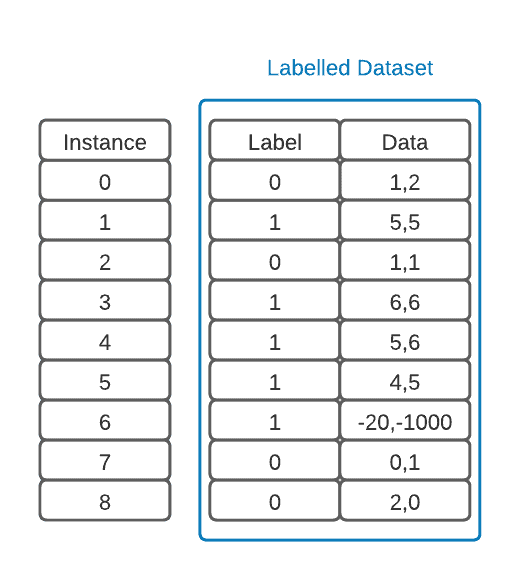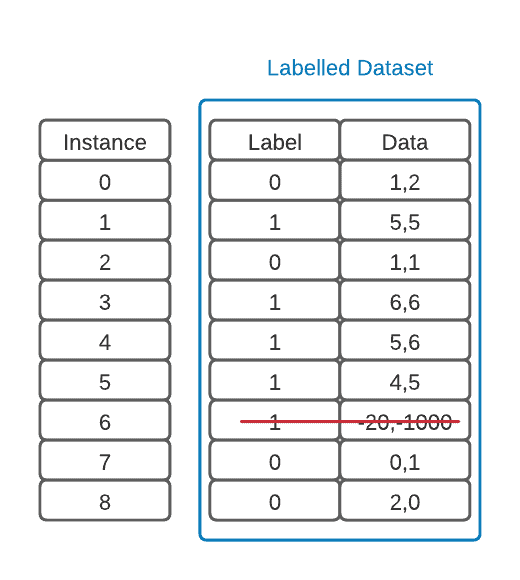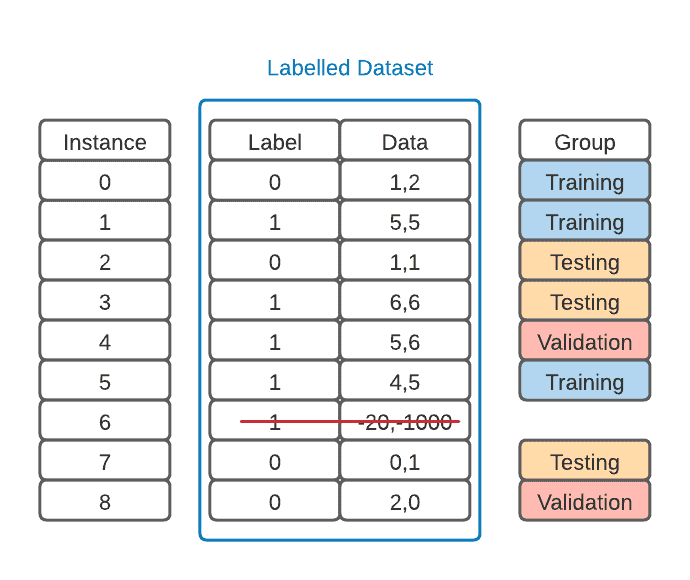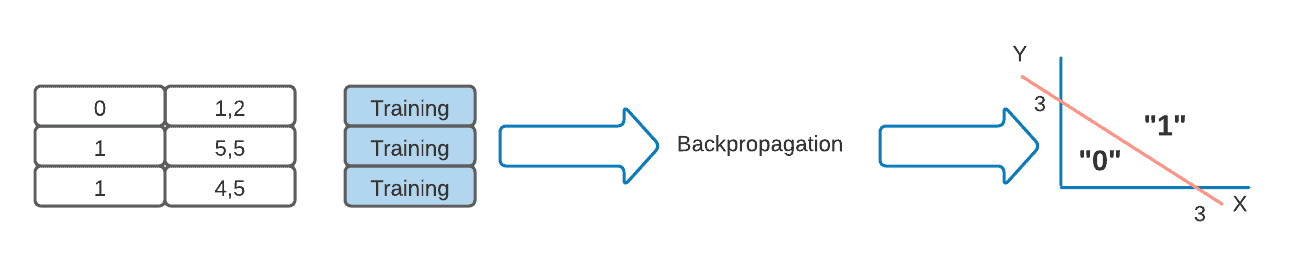## 1. Introduction

In this tutorial, we will discuss the training, validation, and testing aspects of neural networks. These concepts are essential in machine learning and adequately represent the different phases in a model’s maturity. It’s also important to note that these ideas are adjacent to many others, such as cross-validation, batch optimization, and regularization.

## 2. Concepts

The field of machine learning has expanded tremendously thanks to neural networks. These neural nets are employed for a wide variety of reasons because they are very flexible models that can fit almost any kind of data, provided that we have sufficient computing resources to train them in a timely manner. To efficiently exploit these learning structures, we need to make sure that the model generalizes the information that is being processed.

The problem here is that if we feed all the data we have for the model to train, there is no way that we can test if the model has correctly extracted a function from the information. This metric is called accuracy, and it’s essential for assessing the performance of our model.

### 2.1. Training vs. Testing

Alright, we can begin by making a training set and a testing set. We can now train our model and verify its accuracy using the testing set. The model has never seen the test data during training. Therefore, the accuracy result we will obtain will be valid. We can use different techniques to train a neural network model, but the easiest to understand and implement is backpropagation. Now, let’s say that we get a less-than-favorable performance from our training-testing approach. We can maybe change some hyperparameters from our model and try again.

However, if we do so, we will be using the results from the test set to tune the training of the model. There is something wrong with this approach in theory because we are adding a feedback loop from our test set. This will falsify the accuracy results that we will generate because we are changing parameters based on the results we achieve. In other words, we are using the data from the test set to optimize our model.

### 2.1. Purpose of Validation Sets

To avoid this, we perform a sort of “blind test” only at the end. In contrast, to iterate and make changes throughout the development of the model, we use a validation set. Now we can use this validation set to fine-tune various hyperparameters to help the models fit the data. Additionally, this set will act as a sort of index for the actual testing accuracy of the model. This is why having a validation data set is important.

We can now train a model, validate it and change different hyperparameters to optimize performance and then test the model once to report its results.

Let’s see if we can apply this to an example.

## 3. Implementation

To implement these notions in a classic supervised learning fashion, we must first obtain a labeled data set to work with. An example of one with two classes that use coordinates as a single feature will be represented below:The first thing to note is that there is an outlier in our data. It’s good practice to find these using common statistical methods, examine them, and then remove those that don’t add information to the model. This is part of an important step called data pre-processing:### 3.1. Splitting Our Dataset

Now that we have our data ready, we can split it into training, validation, and testing sets. In the figure below, we add a column to our data set, but we could also make three separate sets:We must ensure that these groups are balanced so that our model is less biased. This means that they must have more or less the same amount of examples from each label. Failure in balancing could lead to the model not having enough examples of a class to learn accurately. This could also put the test results in jeopardy.

In this binary classification example, our training set has two “1” labels and only one “0” label. However, our validation set has one of each, and our testing set has two “0” labels and only one “1” label. Because our data is minimal, this is satisfactory.

However, we could change the groups that we defined and pick the best configuration to see how the model performs in testing. This would be called cross-validation. K-fold cross-validation is widely used in ML. However, it’s not covered in this tutorial.

### 3.2. Training and Testing Our Model

Moving on, we can now train our model. In the case of our feed-forward neural net, we could use a backpropagation algorithm to do so. This algorithm will compute an error for each training example and use it to finely adjust the weights of the connections in our neural net. We run this algorithm for as many iterations as we can until it’s just about to overfit our data, and we get the model below:In order to verify the correct training of the model, we feed our trained model the validation dataset and ask it to classify the different examples. This will give us validation accuracy.

If we were to have an error in the validation phase, we could change around any hyper-parameters to make the model perform better and try again. Maybe we can add a hidden layer, change our batch size or even adjust our learning rate depending on the optimization methods.

We can also use the validation dataset for early stopping to prevent the model from overfitting data. This would be a form of regularization. Now that we have a model that we fancy, we simply use the test dataset to report our results, as the validation dataset has already been used to tune the hyper-parameters of our network.

## 4. Conclusion

In this article, we discussed the different notions of training, testing, and validating in machine learning.